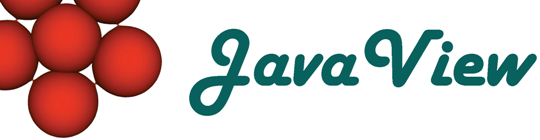Overview  Animation  Classic Surfaces  Parm Surfaces  Curves on Surfaces  Discrete Geodesics  ODE  Platonic Solids  Cycloid  Root Finder  Harmonic Maps  Rivara Bisection  Scalar Field  Weierstrass  Closed Polygon  Elastic Curve  Billiard in an Ellipse  LIC Visualization  Discrete VF  Hodge Splitting  Textured Surface  Surfaces of Rotation  Mean Curvature Flow  Pythagoraen Tree  Julia Sets

## Julia and Mandelbrot Set Explorer

The Mandelbrot set shown to the right determines another set of fractals, so called Julia sets. Each point c in the Mandelbrot set determines a Julia set J(c). Picking and dragging with left mouse in the Mandelbrot image on the right will generate a new Julia set J(c) shown to the left. Mathematically we can say that the Mandelbrot set is the parameter space which describes the entirety of Julia sets.

For a given parameter c the Julia set J(c) is defined as the subset of points z of the complex plane for which the iteration

Iterate[z -> z^2+c]

does not diverge to infinity. Computationally, the algorithm checks for each pixel z if the sequence z,Iterate(z),Iterate(Iterate((z)),... stays within a certain region of the complex plane after a fixed number of iterations. The number of performed iterations can be adjusted using the slider "Number of iterations". The slider "Block Size" allows to work with a coarser pixel grid to speed up computations at the cost of resolution.

When the parameter c lies inside the black region of the Mandelbrot set then the corresponding Julia set has a non-empty black interior.

Some possible interactions:

• Pick inside the Mandelbrot image and see the Julia set being recomputed. The components of the picked complex parameter c is shown.
• Pick inside the Julia image and see the iterates of the picked point under the Julia map.
• Select a rectangular region in display to zoom into image: keep pressing the key 'm' while dragging a rectangle.
• Press the RESET button to initialize the applet, and to zoom out.
• The HUE slider changes the base value of the rainbow colors.

Note, this is applet is mainly a tutorial for studying image handling in JavaView rather than a full-fledged application to study fractals.

View source code:
PaJuliaSet.java of applet
PjJuliaSet.java
of project
PjJuliaSet_IP.java
of info panel.